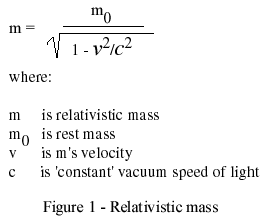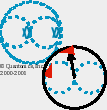Arches

Problematic Einsteinian Relativity Memes
by Doug Renselle

Please consider our work here as 'in progress.' We plan to use this web page to develop memes which apparently put
Einstein's relativity theories in question. Indeed our work here puts physics' use of formal mathematics in question.

"[A] fundamental error is confusion of time and space."
Henri Louis Bergson
Time and Free Will

Page 181."Assume a running jet engine represents quantum reality.
Assume a crescent wrench represents mathematics as an analytical tool.
What happens when we apply mathematics to quantum reality?
Same outcomes as when we throw a crescent wrench into a running jet engine."
Doug - 6Jan2002.

"Relativity implies relationships between [, e.g.,] energy and
momentum [, et al.,] different from those of classical physics."
Page 16, Paradox Lost, by Philip R. Wallace, Springer-Verlag, 1996.
Our brackets.

Wallace's quote here is crucial to understanding our criticisms of Einstein's
own versions of relativity. Our criticisms find their many foci in Einstein's
countless personal and most fundamental classicisms.

Wallace goes on to say in his next sentence, "It [relativity] requires that
energy and momentum be related in essentially the same way as time and space coordinates."
But space is only a measurable in classical physics. It is classically undefined and undefinable.

To paraphrase Bergson, "relativity assumes reality is
both stable and objects in reality are independent of one another."
Zero Point Energy and Quantum Vacuum Flux deny those essential Einsteinian relativity assumptions.
To make our criticisms even more emphatic, Einstein's time is naught but a space-rate proxy.
So Einstein's relativity requires that we relate notions of reality in terms of invalid
classical space-time "measurables" one of which is 'defined' in terms of its 'scalar' proxy.

So, we can infer that Einstein's notions of space and time are simply
wrong according to Millennium III understandings of quantum reality.
Indeed he was!
His classical view of energy assumes that energy is proportional to continuous
'change' amplitude. But in quantum reality, energy is proportional, n¤t
to amplitude, but to quantized/fretted/latched frequency.

(Viz. an atom as a guitar/violin with one string per electron and each string
latched/fretted at that electron's stable frequency. Photons of appropriate
energies can play said violin's highest frequency notes to limited extents.
Then imagine ensembles of atomic guitars and violins: QED!)
Doug - 13Mar2003.

Discomfiting aspects of Einstein's relativity, i.e., concepts which still are personally problematic for Doug Renselle:

1.

Figure 1 parametrics require three fundamental physical concepts: mass, length, and time. All of these physical concepts are said to be 'measurable.' None are definable. Is it important that we define mass, length, and time in terms of a more fundamental concept? If you answer "yes," what is that more fundamental concept? What are mass, length, and time defined in terms of that more fundamental concept? If you answer "no," please explain why? What assumptions are you making which permit us to base our entire scientific enterprise on measurables? Are your assumptions based upon reality? Is a physics based upon undefinable measurables real? How do you know? Is reality physical?

See our November, 2002 Einstein Wrong? on Inverse Quantum Zeno timings.

Figure 1 parametrics depend upon several mathematical concepts: objective formalism, analytic calculus, parametric independence, identity, etc.

2.

Einstein's theories of relativity depend on an assumption that certain physical conditional identities exist.

Our concern is: As shown in Figure 1 above, Einstein imposed concepts of mathematical identity on physical reality. When one does this, one apparently assumes:

 that specific physical measurables and combinations of other measurable phenomena may be identical and vice versa, or that physical entities may be identical to combinations of other physical entities and vice versa, or etc. Here, we see that a concept of physical identity depends further upon a concept of objective reducibility. Indeed, a concept of identity insinuates a legion of other objective concepts, e.g., analyticity, homogeneity, independence (i.e., lisr), state-icity, immutability, zero momentum measurement, et al.

Figure 1, above shows an equal sign forming an identity twixt left side of 'equation' and right side of equation.

Figure 1, above shows a difference in equation's denominator which assumes several identity conditions: v identical c, when identical v/c is 'one,' 1 identical 1, and identical 1 minus identical 1 produces zero.

3.

Einstein assumed that '1' (one) may be used to represent physical reality (I.e., e.g., in his relativistic equation for mass, shown in Figure 1.)

Our concern is: what does '1' represent physically? No two physical objects are identical, so how can we demonstrate valid oneness by a ratio of one exact physical object to another as '1?'

Just what is '1' physically? Define what one is physically.

If 'one' is a ratio of two physical entities or measurements which are supposed to be equal, we know that is not possible since all physical entities are quantons and thus subject to Heisenberg's uncertainty principle. Two uncertain entities ratioed against each other will rarely have a probability of 'one' as their ratio. Einstein's relativistic mass equation depends upon physical measurements holding still (i.e., 'zero momentum') to produce an ideal 'one' ratio which can then be differenced with some mathematically ideal '1' which apparently does not exist.

Furthermore, and this is perhaps most problematic, classical '1s' may be classically-mechanically, canonically ratioed and canceled. Quantum '1s' may n¤t be ratioed and canceled classically-mechanically. Where classicists assume classical one has classical 'state,' i.e., perpetual duration as immutable stoppability, quantumists must assume that a quantum one is flux itself, i.e., a packet of quanta, unstoppable and perpetually, quantum~durably evolving. See quantonics' memeos of cancel, duration, evolution, negative, objective, perpetual~motion, positive, subjective, truth, etc.

Here is material from our August, 2000 News which shows graphically what we intend:

Our Quantonic heuristic is that quantum nature offers us no physi of either oneness or zeroness. Certainly, nature offers us no physi of identity! Ditto infinity, tautology, etc.

"Doug, how can you say that?"

Look at our Quantonic representation of a nonphysically expressible '1' below:

 '1'August 2000 Figure 2
-
Quantonics' quantum 'one' as inexpressible physically.
(How could we change this graphic
to make it expressible physically?

Quantum reality's best opportunity to express a physical 'one' is shown in Figure 2. But it cannot do that. Right side numerator and denominator quantons are stochastically never 'same.' They will always differ by at least a Planck quantum uncertainty.

From a quantum perspective an unchanging, immutable, ideal '1' (one) is a physical impossibility. All physical ratios are Planck rate uncertainty interrelationships.

4. Einstein assumed that '0' (zero) may be used to represent physical reality (I.e., e.g., in his relativistic equation for mass.)

Our concern is: what does '0' represent physically? No two physical objects are identical, so how can we demonstrate valid physical zeroness by a difference between two exact physical objects?

Just what is '0' physically? Define what zero is physically.

Similar to our discussion for 'one' above, if zero is a difference of two physical entities or measurements which are supposed to be equal, we know that is not possible since all physical entities are quantons and thus subject to Heisenberg's uncertainty principle. Two uncertain entities differenced will rarely have a probability of 'zero' as their difference. Einstein's relativistic mass equation depends upon physical measurements holding still to produce an ideal 'zero' difference.

From a quantum perspective an unchanging, immutable, ideal '0' (zero) is a physical impossibility. All physical differences are Planck rate uncertainty interrelationships.
5.

Einstein assumed that light's velocity is absolute. We need to ask, mimicking Fred Alan Wolf, "Herr Einstein, what is light's velocity to a photon? From individual photons' perspectives do different photons have differing light velocities? Or, to all photons, is their velocity 'zero' or some other measurable? Did not you say that light's speed is absolute—ubiquitously? Or do you mean to imply that, as Wheeler and Feynman conjectured, there is only one photon?"

What fatal and flawed assumption did Einstein make? He assumed reality is OGC, One Global Context. As a result, he applied classical unilogical/homological mathematics to physical problems. He assumed reality has only one unilogical time frame. He assumed reality's—assumed one global—time is homogeneous. He assumed one time fits all reality's constituents.

Quantum reality shows us that all of reality's classical measurables (masses, lengths, times, and gravities) and everything else in actuality are not homogeneous! Rather, they are heterogeneous! Let's compare classical and quantum models of reality:

 Classical reality is a reality of one pre-existing, closed monolith whose separable 'objects' change/move via a drum beat of one homogeneous time. (vis. 'cathedral.') As Mae-wan Ho interpreted Henri Bergson (paraphrased), "...an infinitely divisible, quantitative, homogeneity." Quantum reality is a reality of many evolving, open autonomies each with their own rhythms, all quantum-cohesively commingling and compenetrating. (vis. 'bazaar.') As Mae-wan Ho interpreted Henri Bergson (paraphrased), "...an indivisible, qualitative, heterogeneity."

Einstein would accept former and deny latter.

These same classical assumptions, which Einstein made, drove his conclusions that, "God does not throw dice, and that quantum theory is absurd, i.e., e.g., action at a distance is absurd."

6.

For general relativity Einstein assumed that any geometrical interval remained invariant across continuous coordinate transformations. This is how Einstein retained classical objectivity in GR.

But what else is Einstein assuming here?

He assumes that an invariant interval is an objective interval between any two points. In order for that interval to remain invariant, its points have to hold still for arbitrary time, assuming that transformations in physical reality consume time.

But quantum reality doesn't hold still. Einstein's points cannot hold still. His classically objective invariant geometric interval is impossible in quantum reality! GR loses its objectivity.

Quantum relativity is subjective!

Doug.

To contact Quantonics write to or call:

Doug Renselle
Quantonics, Inc.
Suite 18 #368 1950 East Greyhound Pass
Carmel, INdiana 46033-7730
USA
1-317-THOUGHT

©Quantonics, Inc., 2000-2023 — Rev. 18May2013  PDR — Created 31Jul2000  PDR
(2Aug2000 rev - Correct 'back' links.)
(4Sep2000 rev - Correct misspelling.)
(12Oct2000 rev - Add item on Einstein's assumptions re
: absolute light velocity, and one homogeneous time frame.)
(12Oct2000 rev - Copy example of a physical, quantum '1' from our August, 2000 News.)
(11Nov2001 rev - Add Bergson top of page quote.)
(6Dec2001 rev - Add top of page frame-breaker.)
(7Jan2002 rev - Add a top of page Doug quote.)
(2Jun2002 rev - Add link to our newly completed review of Bergson's Time and Free Will.)
(11Jun2002 rev - Add specific, topic 33, p. 181 link just below above change.)
(23Jul2002 rev - Change QELR links to A-Z pages.)
(16Nov2002 rev - Add item 1 link to our QSBP&P Einstein Wrong.)
(13Mar2003 rev - Add page top Wallace quote in red text.)
(14Oct2003 rev - Do a spell check and correct typos and spelling mistakes.)